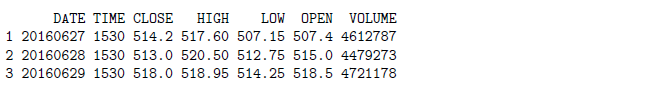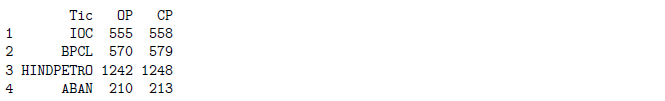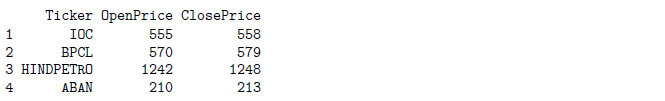# R Weekly Bulletin Vol – XIIThis week’s R bulletin will cover topics on how to resolve some common errors in R. Hope you like this R weekly bulletin. Enjoy reading!

### Shortcut Keys

1. Find and Replace - Ctrl+F
2. Find Next - F3
3. Find Previous - Shift+F3

### Problem Solving Ideas

#### Resolving the ‘: cannot open the connection’ Error

There can be two reasons for this error to show up when we run an R script: 1) A file/connection can’t be opened because R can’t find it (mostly due to an error in the path) 2) Failure in .onLoad() because a package can’t find a system dependency

Example:

symbol = "AXISBANK"
noDays = 1
dirPath = paste(getwd(), "/", noDays, " Year Historical Data", sep = "")
fileName = paste(dirPath, symbol, ".csv", sep = "")
data = as.data.frame(read.csv(fileName))

Warning in file(file, "rt"): cannot open file 'C:/Users/Madhukar/Documents/ 1 Year Historical DataAXISBANK.csv': No such file or directory Error in file(file, "rt"): cannot open the connection

We are getting this error because we have specified the wrong path to the “dirPath” object in the code. The right path is shown below. We missed adding a forward slash after “Year Historical Data” in the paste function. This led to the wrong path, and hence the error.

dirPath = paste(getwd(),"/",noDays," Year Historical Data/",sep="")

After adding the forward slash, we re-ran the code. Below we can see the right dirPath and fileName printed in the R console.

Example:

symbol = "AXISBANK"
noDays = 1
dirPath = paste(getwd(), "/", noDays, " Year Historical Data/", sep = "")
fileName = paste(dirPath, symbol, ".csv", sep = "")
print(head(data, 3))#### Resolving the ‘could not find function’ Error

This error arises when an R package is not loaded properly or due to the misspelling of the function names.

When we run the code shown below, we get a “could not find the function ymd” error in the console. This is because we have misspelled the “ymd” function as “ymed”. If we do not load the required packages, this will also throw up a “could not find function ymd” error.

Example:

# Read NIFTY price data from the csv file
dates = ymed(df$DATE) Error in eval(expr, envir, enclos): could not find function "ymed" #### Resolving the “replacement has” Error This error occurs when one tries to assign a vector of values to an existing object and the lengths do not match up. In the example below, the stock price data of Axis bank has 245 rows. In the code, we created a sequence “s” of numbers from 1 to 150. When we try to add this sequence to the Axis Bank data set, it throws up a “replacement error” as the lengths of the two do not match. Thus to resolve such errors one should ensure that the lengths match. Example: symbol = "AXISBANK" ; noDays = 1 ; dirPath = paste(getwd(),"/",noDays," Year Historical Data/",sep="") fileName = paste(dirPath,symbol,".csv",sep="") df = as.data.frame(read.csv(fileName))# Number of rows in the dataframe "df" n = nrow(df); print(n);# create a sequence of numbers from 1 to 150 s = seq(1,150,1)# Add a new column "X" to the existing data frame "df" df$X = s
print(head(df,3))
Error in $<-.data.frame(tmp, “X”, value = c(1, 2, 3, 4, 5, 6, 7, : replacement has 150 rows, data has 245 ### Functions Demystified #### do.call function The do.call function is used for calling other functions. The function which is to be called is provided as the first argument to the do.call function, while the second argument of the do.call function is a list of arguments of the function to be called. The syntax for the function is given as: do.call (function_name, arguments) Example: Let us first define a simple function that we will call later in the do.call function. numbers = function(x, y) { sqrt(x^3 + y^3) }# Now let us call this 'numbers' function using the do.call function. We provide the function name as # the first argument to the do.call function, and a list of the arguments as the second argument. do.call(numbers, list(x = 3, y = 2))  5.91608 #### rename function The rename function is part of the dplyr package, and is used to rename the columns of a data frame. The syntax for the rename function is to have the new name on the left-hand side of the = sign, and the old name on the right-hand side. Consider the data frame “df” given in the example below. Example: library(dplyr) Tic = c("IOC", "BPCL", "HINDPETRO", "ABAN") OP = c(555, 570, 1242, 210) CP = c(558, 579, 1248, 213) df = data.frame(Tic, OP, CP) print(df)# Renaming the columns as 'Ticker', 'OpenPrice', and 'ClosePrice'. This can be done in the following # manner: renamed_df = rename(df, Ticker = Tic, OpenPrice = OP, ClosePrice = CP) print(renamed_df)#### lapply function The lapply function is part of the R base package, and it takes a list “x” as an input, and returns a list of the same length as “x”, each element of which is the result of applying a function to the corresponding element of X. The syntax of the function is given as: lapply(x, Fun) where, x is a vector (atomic or list) Fun is the function to be applied Example 1: Let us create a list with 2 elements, OpenPrice and the ClosePrice. We will compute the mean of the values in each element using the lapply function. x = list(OpenPrice = c(520, 521.35, 521.45), ClosePrice = c(521, 521.1, 522)) lapply(x, mean)$OpenPrice  520.9333
$ClosePrice  521.3667 Example 2: x = list(a = 1:10, b = 11:15, c = 1:50) lapply(x, FUN = length)$a  10
$b  5$c  50